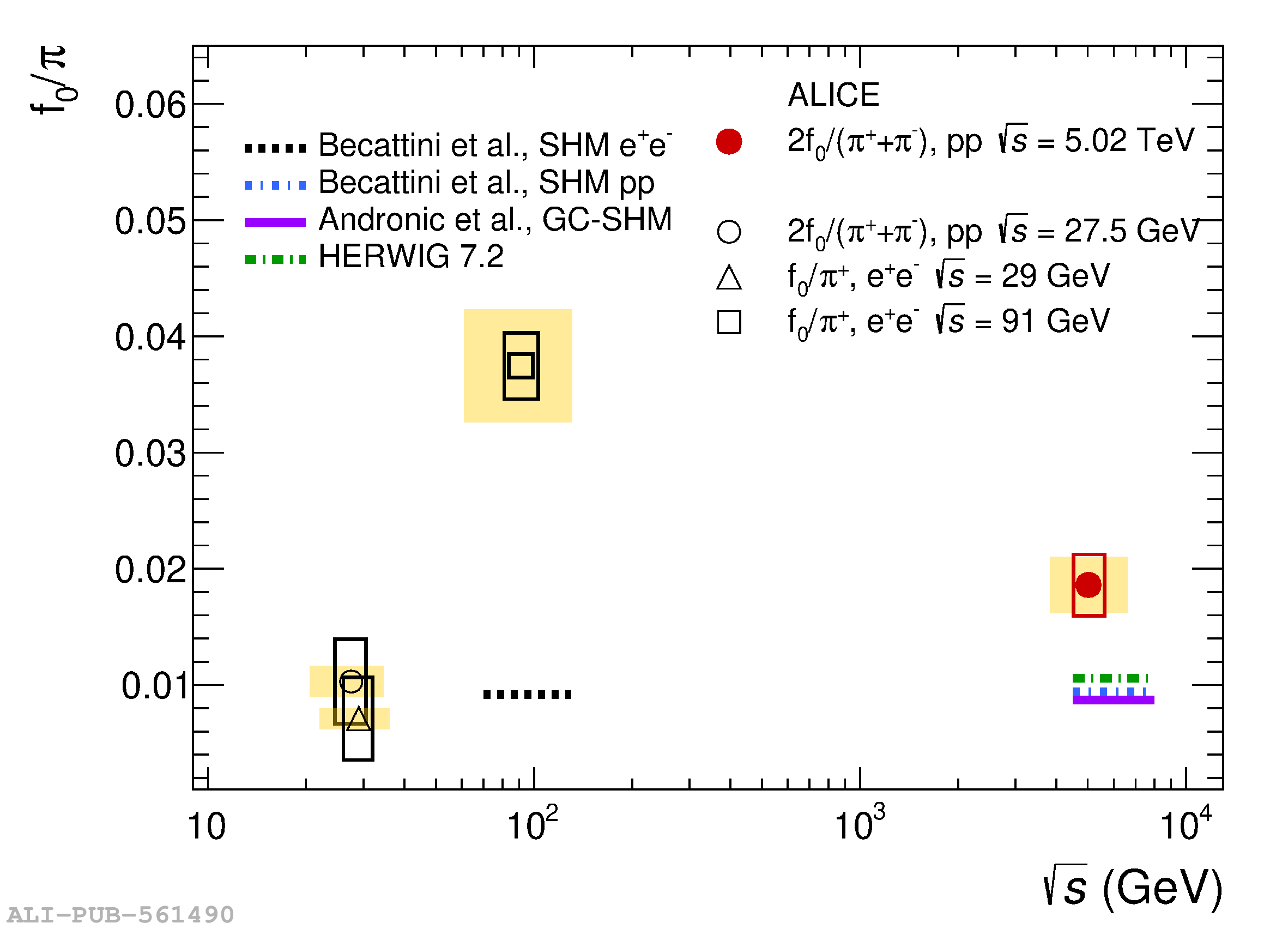# Figure 4

 Comparison of the measured $2\fzeroshort/(\pi^{+}+\pi^{-})$ ratio with measurements in \ee collisions at $\sqrt{s}$ = 29 \GeV , $\sqrt{s}$ = 91 \GeV  and in pp collisions at $\sqrt{s}$ = 27.5 \GeV . The ratios are compared to predictions from statistical hadronisation model (SHM) calculations for \ee collisions  and pp collisions , GC-SHM  and HERWIG 7.2 . The hollow boxes represent the total uncertainty on data. The relative uncertainty of 13\% due to the branching ratio correction  applies to all data points and is shown as a yellow box All error boxes are drawn with an arbitrary horizontal width for visibility.